+
Common Core: S.ID.4

# 68-95-99.7 Rule

Author: Sophia Tutorial
##### Description:

Identify the percent of data that is between two values using a given standard deviation, mean, and the 68-95-99.7 rule.

(more)

Sophia’s self-paced online courses are a great way to save time and money as you earn credits eligible for transfer to many different colleges and universities.*

No credit card required

37 Sophia partners guarantee credit transfer.

299 Institutions have accepted or given pre-approval for credit transfer.

* The American Council on Education's College Credit Recommendation Service (ACE Credit®) has evaluated and recommended college credit for 32 of Sophia’s online courses. Many different colleges and universities consider ACE CREDIT recommendations in determining the applicability to their course and degree programs.

Tutorial
what's covered
This tutorial will explain the 68-95-99.7 rule. Our discussion breaks down as follows:

1. The 68-95-99.7 Rule
2. Finding More Percents

## 1. The 68-95-99.7 Rule

The 68-95-99.7 rule applies to normal distributions and states that 68% of all data points fall within one standard deviation of the mean, 95% of all data points fall within two standard deviations of the mean, and 99.7% of all data points fall within three standard deviations of the mean.

To understand this rule, let's start with the normal distribution. As you can see below, the normal distribution is single peaked and symmetric. Thus, this distribution can be described exclusively by its mean and standard deviation.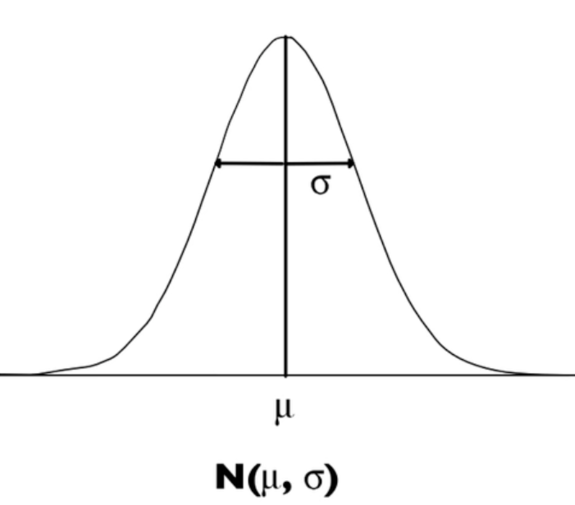All normal curves, all normal distributions, look the same, and exactly like this. The only thing that might make these distributions look a little different is that they may have a wider standard deviation or be centered at a different place. However, since that is all the difference, we can describe the distribution with the notation above (N, mean, standard deviation).

When you learned to calculate standard deviations, you were taught that a good amount of the data points would fall within one standard deviation of the mean. In a normal distribution, you get a good amount of the data points in the first standard deviation.

How many of the data points will fall in the first standard deviation? 68% of all the data falls within the first standard deviation of the mean, from one standard deviation below the mean to one standard deviation above the mean.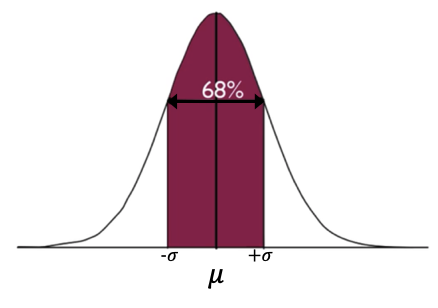If you go out another standard deviation, so now you are two standard deviations below the mean to two standard deviations above the mean, you get 95% of the data points.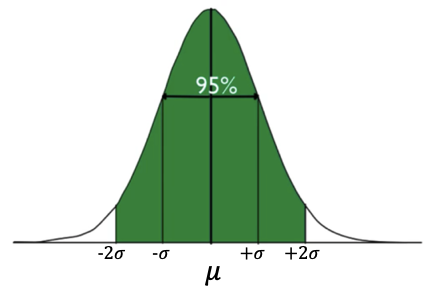If you go all the way out to three standard deviations below the mean and three standard deviations above, you get almost the entire set of data: 99.7%.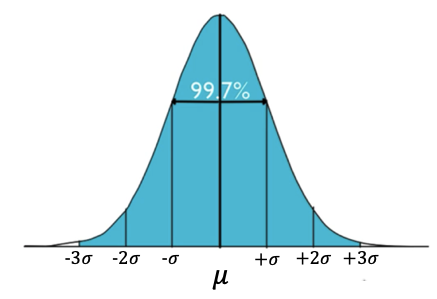This is why it's called the 68-95-99.7 Rule.

• About 68% of the data values are within one standard deviation of the mean.
• About 95% are within two standard deviations above or below the mean.
• About 99.7% of the data, almost all the data, fall within three standard deviations of the mean.
term to know

68-95-99.7 Rule
A rule that applies to normal distributions, stating that 68% of all data points fall within one standard deviation of the mean, 95% of all data points fall within two standard deviations of the mean, and 99.7% of all data points fall within three standard deviations of the mean.

## 2. Finding More Percents

Because of the symmetry of the normal distribution, you can examine this rule further.

You can say that 68% of the data falls within one standard deviation, but because of the symmetry, 34% falls between one standard deviation below and the mean, and another 34% falls between the mean and one standard deviation above.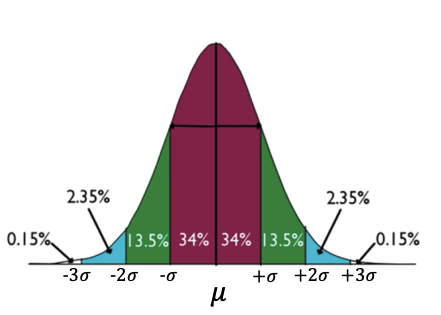You can continue with this logic: the green bars--between one and two standard deviations below and above the mean--each contain 13.5%. That 13.5% is obtained because we know that the two standard variations on each side of the mean contain 95% of the data points. Since 68% is within the red portion--one standard deviation above and below the mean--then the remaining 27% (95-68) must fall within the two green bars. Also, because they have the same area, they must each contain half of that remainder or 13.5%.

Using that same logic again, you can see that about 2.35% of the data points fall within the blue bars.

Way out in the tails, you get almost none of the data points. To make up the full 100%, another 0.15% falls within each of those tails further out than three standard deviations away.

EXAMPLE

A particular battery from a company has a lifetime that is normally distributed with a mean of 500 hours, and a standard deviation of 18 hours. What percent of batteries last between 482 and 518 hours?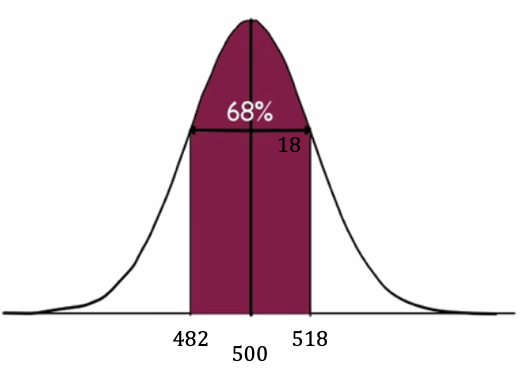482 and 518 are exactly one standard deviation above and below the mean of 500. Using the 68-95-99.7 rule, we can say that 68% of batteries last between 482 and 518 hours.

EXAMPLE

What percent of batteries from this company last between 446 hours, and 536 hours?

Well, 446 is three standard deviations below the mean. 536 is two standard deviations above the mean. So, there are two ways to calculate the answer to this question.

1. You could calculate it as the full 95% percent (the green area), plus the 2.35% (one of the blue bars).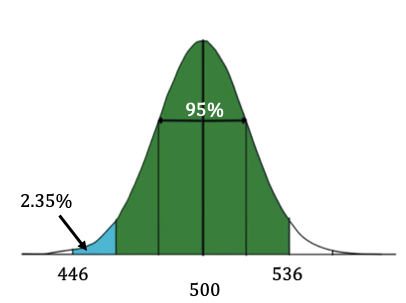95% + 2.35% = 97.35%

2. You could have also calculated it as 99.7% (all of the blue area) and subtracted the extra 2.35% (the blue bar to the far right).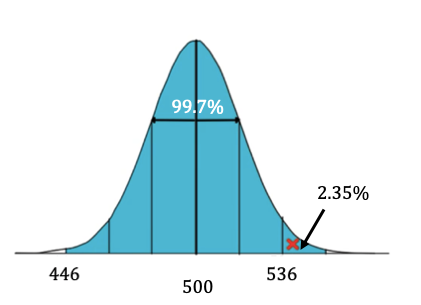99.7% - 2.35% = 97.35%

Either way, you end up with 97.35%.

EXAMPLE

What percent of batteries last longer than 464 hours?
464 is two standard deviations below the mean, so there are a few different ways to do this calculation.

1. You could just start adding each area above 464.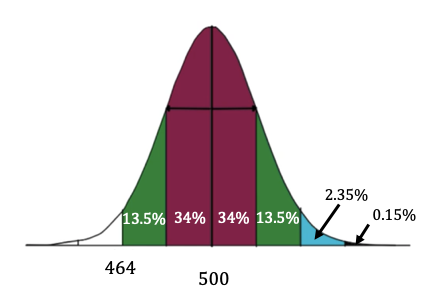13.5% + 34% + 34% + 13.5% + 2.35% + 0.15% = 97.5%

2. You could add the 95% plus the remaining 2.5% in the upper tail.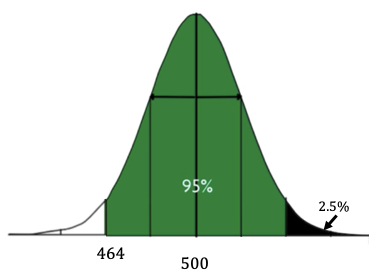95% + 2.5% = 97.5%

3. The entire curve is 100%, and the only part you don't want is the 2.5% on the left.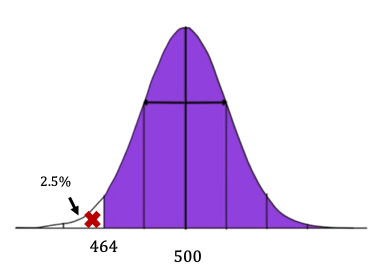100% - 2.5% = 97.5%

However you calculate this, you should end up with the same answer: 97.5% of batteries last longer than 464 hours.

summary
The 68-95-99.7 Rule is a way to generate approximate percents of values that will be within a particular interval of the normal distribution. You can combine this rule with your knowledge of the symmetry of the normal distribution to find more percents than just 68, 95, and 99.7. This rule will not work if the values are not at integer standard deviations, meaning whole numbers of standard deviations away from the mean.

Good luck!

Source: Adapted from Sophia tutorial by Jonathan Osters.

Terms to Know
68-95-99.7 Rule

A rule that applies to normal distributions, stating that 68% of all data points fall within one standard deviation of the mean, 95% of all data points fall within two standard deviations of the mean, and 99.7% of all data points fall within three standard deviations of the mean.

Rating